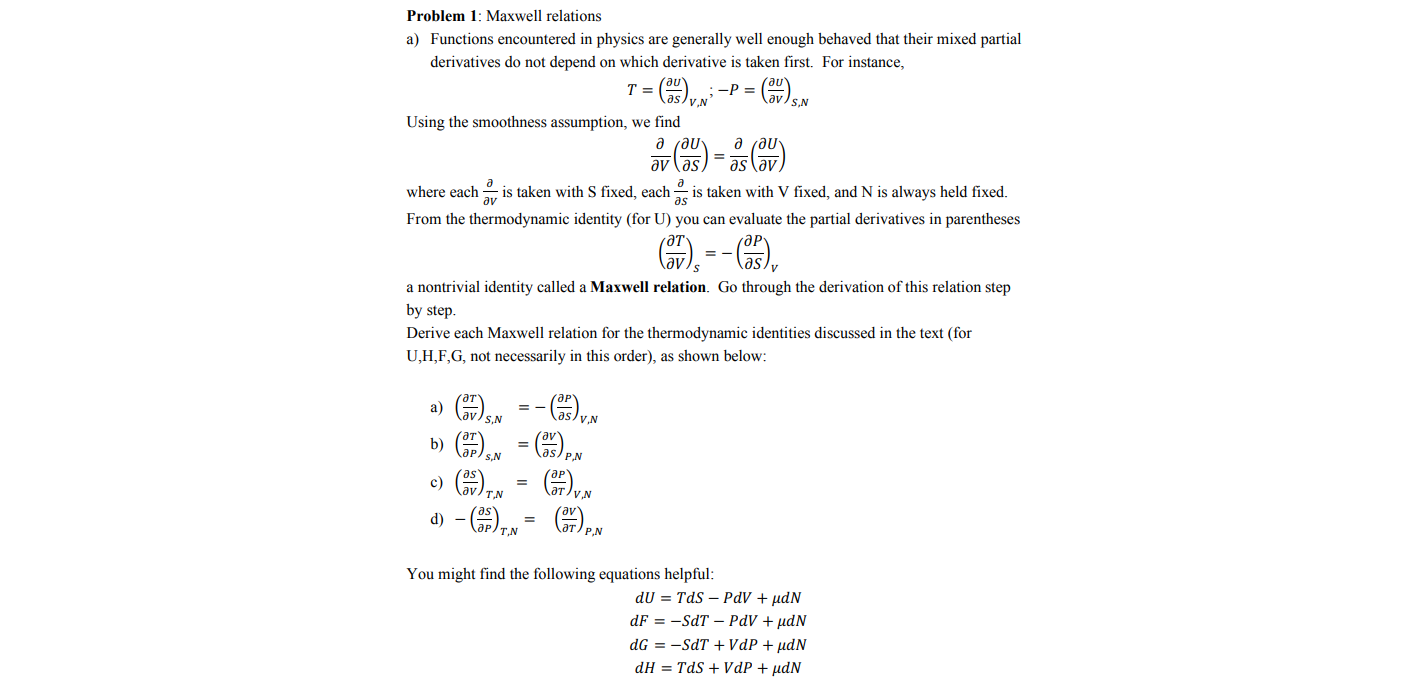# Problem 1: Maxwell relations a) Functions encountered in physics are generally well enough behaved that their mixed partial derivatives do not depend on which derivative is taken first. For instance, -P = aslvN S,N Using the smoothness assumption, we find д гди- where each av is taken with S fixed, each is taken with V fixed, and N is always held fixed. From the thermodynamic identity (for U) you can evaluate the partial derivatives in parentheses a nontrivial identity called a Maxwell relation. Go through the derivation of this relation step by step. Derive each Maxwell relation for the thermodynamic identities discussed in the text (for U,H,F,G, not necessarily in this order), as shown below: a) lav S,N as/y.N b) s/ P.N N*() d) - (), c) ат/у av ат/ р N You might find the following equations helpful: dU = TdS – PdV + µdN dF = -SdT – PdV + µdN dG = -SdT + VdP + µdN dH = TdS + VdP + µdN

Questionhelp_outlineImage TranscriptioncloseProblem 1: Maxwell relations a) Functions encountered in physics are generally well enough behaved that their mixed partial derivatives do not depend on which derivative is taken first. For instance, -P = aslvN S,N Using the smoothness assumption, we find д гди- where each av is taken with S fixed, each is taken with V fixed, and N is always held fixed. From the thermodynamic identity (for U) you can evaluate the partial derivatives in parentheses a nontrivial identity called a Maxwell relation. Go through the derivation of this relation step by step. Derive each Maxwell relation for the thermodynamic identities discussed in the text (for U,H,F,G, not necessarily in this order), as shown below: a) lav S,N as/y.N b) s/ P.N N*() d) - (), c) ат/у av ат/ р N You might find the following equations helpful: dU = TdS – PdV + µdN dF = -SdT – PdV + µdN dG = -SdT + VdP + µdN dH = TdS + VdP + µdN fullscreen